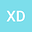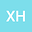Ground states for critical fractional Schr\”{o}dinger-Poisson systems with vanishing potentials
••• Xilin Dou,
• xiaoming he
Xilin Dou
Minzu University of China

Corresponding Author:[email protected]

Author Profilexiaoming he
Minzu University of China
Author Profile## Abstract

This paper deals with a class of fractional Schr\”{o}dinger-Poisson system $\begin{cases}\displaystyle (-\Delta )^{s}u+V(x)u-K(x)\phi |u|^{2^*_s-3}u=a (x)f(u), &x \in \R^{3}\\ (-\Delta )^{s}\phi=K(x)|u|^{2^*_s-1}, &x \in \R^{3}\end{cases}$with a critical nonlocal term and multiple competing potentials, which may decay and vanish at infinity, where $s \in (\frac{3}{4},1)$, $2^*_s = \frac{6}{3-2s}$ is the fractional critical exponent. The problem is set on the whole space and compactness issues have to be tackled. By employing the mountain pass theorem, concentration-compactness principle and approximation method, the existence of a positive ground state solution is obtained under appropriate assumptions imposed on $V, K, a$ and $f$.
21 Dec 2021Submitted to Mathematical Methods in the Applied Sciences
22 Dec 2021Submission Checks Completed
22 Dec 2021Assigned to Editor
27 Dec 2021Reviewer(s) Assigned
08 Mar 2022Review(s) Completed, Editorial Evaluation Pending
11 Mar 2022Editorial Decision: Revise Minor